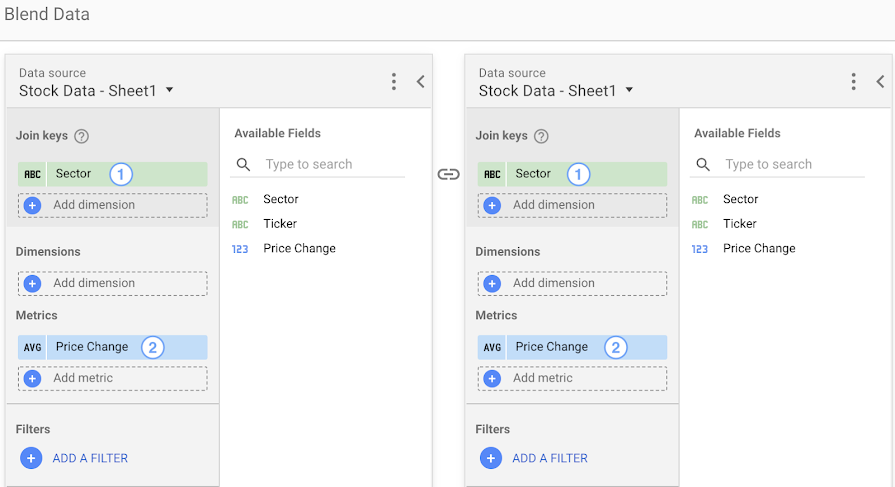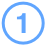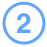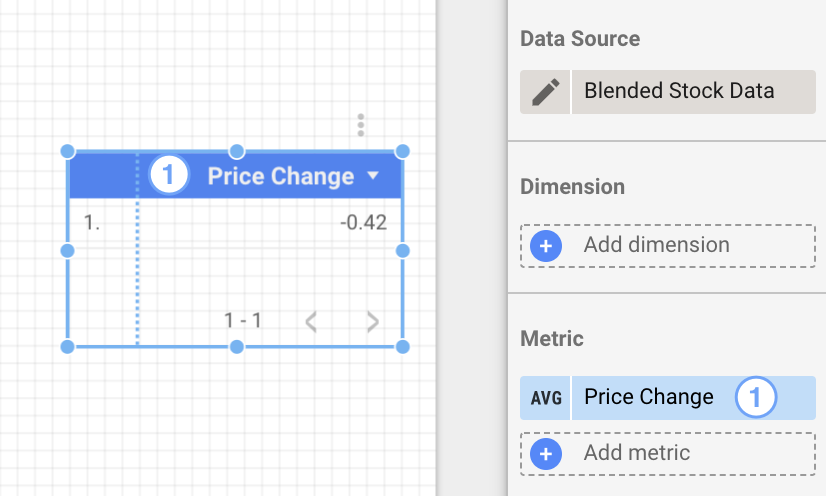# Use blending to reaggregate data

Learn how to work with previously aggregated data.

Reaggregation is a common need in data visualization. This article will help you understand the concept of reaggregation and how to achieve it in Looker Studio using data blending.

One example of reaggregation is calculating the average of averages. For example, say you have a table of stock price changes:

 Sector Ticker Price Change Tech GOOG +6 Tech AAPL +5 Tech MSFT -3 Tech NFLX -1 Energy E1 +2 Energy E2 +10 Energy E3 -3 Finance F1 -6

The average price change for this data is a simple aggregation.

 Average of Price Change 1.25

To calculate the average price change for every sector, you'd group this table by the Sector dimension.

 Sector Average of Price Change Tech 1.75 Energy 3 Finance -6

To reaggregate this data, you'd apply another aggregation function, for example, applying average again:

 Average of Average of Price Change -0.42

## Reaggregation in Looker Studio

To reaggregate metrics in Looker Studio, use data blending. Blending lets you work around the fact that previously aggregated fields are set to the AUTO field type. You can't change this field type, nor can you apply another aggregation function to such fields.

For example, to find the average change of stock prices per sector in Looker Studio, you'd create a blend configuration that joins the same data source with itself. Use Sector as the join key, and include the Average Price Change metric in both the left and right data sources, as shown below:SectorAverage Price Change

This blended data source lets you apply new aggregations on the previously aggregated Price Change field.

### Blending disaggregates data

Blending data creates a new table from the columns that you select in the blend configuration. Metrics in the new table are treated as unaggregated numbers.

Because Price Change is no longer an aggregated metric, you can now apply a new aggregation function on it. The table below shows the results of creating a new metric AVG(Price Change) with the previously aggregated numbers:Price Change

This new metric reaggregates the numbers 1.75, 3 and -6 and displays their average: -0.42 .

## Create a ratio column using blending

Another use for blending is to create ratio metrics with already aggregated numbers. Say you want to create a ratio column that divides one metric by another.

In this example, we'll use two fields; Clicks and Impressions, coming from two different data sources.

You can create a ratio column with a calculated field Clicks/Impressions by blending these two data sources.

 Website Clicks Impressions Clicks / Impressions google.com 300 2000 0.15 facebook.com 400 2500 0.16 twitter.com 200 2000 0.1 Grand Total 900 6500 0.41

All the rows of Clicks/Impressions have correct information except the summary row which shows the sum of the ratio column `SUM(Clicks / Impressions)`. This happens because Clicks/Impressions is calculated for each row [0.15, 0.16, 0.1] and then the `SUM` function is applied to it. [0.15 + 0.16 + 0.1 = 0.41].

The correct result is 900/6500 = 0.14 .You can do this by calculating the ratio column values using the formula `SUM(Clicks) / SUM(Impressions)`.

 Website Clicks Impressions SUM(Clicks) / SUM(Impressions) google.com 300 2000 0.15 facebook.com 400 2500 0.16 twitter.com 200 2000 0.1 Grand Total 900 6500 0.14

In this case, the summary row shows `SUM( SUM(Clicks) / SUM(Impressions) )`. `SUM(Clicks)`  is divided by `SUM(Impressions)` to give 0.14. The `SUM` function is then applied to it again. The result is still 0.14.